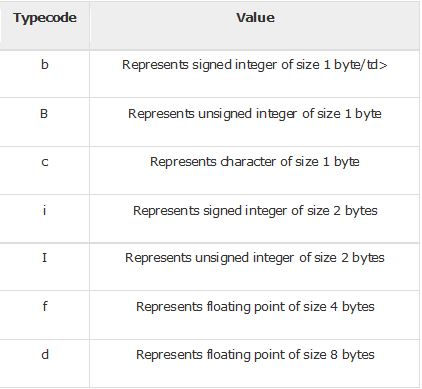Special Offer - Enroll Now and Get 2 Course at ₹25000/- Only Explore Now!# Arrays in Python

July 17th, 2019

## Arrays in Python

An array is a collection of elements that are of the same data type.  Arrays are used for storing multiples values in a single variable. An array is very useful if you need to have a list of items that you want to loop through. You can also group similar items within a single array.
Without an array, you would have to create a separate variable for each value and specifically call each variable which can be quite cumbersome. With arrays, you can store all those lists of values in a single variable and access the values by referring to its index number.
In Python, the “array” data structure in core Python is not as efficient or reliable so when people talk about arrays, they usually mean lists. A list is basically a data structure in Python which is quite similar to an array. A list can contain data types like Integers, Strings, and Objects. It can also contain values of different data types in a single list variable.
Lists and arrays are mutable and therefore, can be modified even after it has been created. Python does provide NumPy array which are grids containing values used in data science. NumPy arrays are used to store numerical lists of data and for representing vectors and tensors. However, they do not support string values. Here, we will be looking into both lists and arrays in Python to get a better understanding.

### Defining an array in Python

After importing the array module, you can define an array by assigning a suitable variable name.  Arrays use type codes which are used to define the type of value that a particular array will hold. Below are some of the common type codes.```from array import * array_list = array('i', [2, 5, 1, 5, 7, 3, 4]) print(str(array_list)) num_list = array_list.tolist() print(num_list) ```
##### Output
 ```Array('i', [2, 5, 1, 5, 7, 3, 4])                                       [2, 5, 1, 5, 7, 3, 4]  ```

### Accessing an array element in Python

Any element within an array can be accessed by referring to is index value.

 ```from array import * array_list = array('i', [10,20,30,40,50]) print (array_list) print (array_list)```
##### Output
 ```10 30 ```

### Looping through arrays in Python

A for in loop can be used to loop through the values within an array.

 ```from array import * array_list = array('i', [10,20,30,40,50]) for x in array_list: print(x)```
##### Output
 ```10 20 30 40 50 ```

### Adding more items to an array in Python

Similar to a list, the insert() method can be used to add more items to an array. You can also choose the position or index in which you want to place the item.

 ```from array import * array_list = array('i', [10,20,30,40]) array_list.insert(1,60)#here, 1 refers to the position where the value 60 would be placed for x in array_list: print(x) ```
##### Output
 ```10 60 20 30 40 ```

### Updating an array in Python

An existing element can be updated or modified by specifying the index of the particular item to be updated.

 ```from array import * array_list = array('i', [10,20,30,40,50]) array_list = 70 for x in array_list: print(x) ```
[/su_table]
##### Output
 ```10 20 30 70 50 ```

### Removing an item from an array in Python

An item within an array can be removed by using the remove() function where the specific value to be removed is mentioned within the brackets.

 ```from array import * array_list = array('i', [10,20,30,40,50]) array_list.remove(20) for x in array_list:  print(x) ```
##### Output
 ```10 30 40 50 ```

### Performing arithmetic computation in a Python array

A Python array can be very useful for directly performing arithmetic computations on a large set of data. The following example shows an array which is divided by 4. The output shows that all the values within the array are each divided by 4.

 ```from numpy import array num_array = array([24, 12, 504, 1224]) division = num_array/4 print(division) ```
##### Output
 ```[   6.    3.  126.  306.]        ```

### Searching an element in a Python array

You can search for a specific element inside an array by using the in-build index method which specifies the element you are looking for with enclosed brackets as shown below

 ```from array import * array_list = array('i', [10,20,30,40,50]) print (array_list.index(50)) ```
##### Output
 ```4 #so the element “50” can be found in index 4 ```

### Conclusion

Arrays and lists are similar as they are both used to store multiple values in a single variable. However, they both have a different purpose as well. Arrays are primarily used for arithmetic computations and data science. They can store only one data type and have a fixed size. For doing large numerical computations, NumPy arrays are best. On the other hand, a list is more flexible as it allows values of different data types which can all be stored in a single list variable. It also allows items to be easily appended so it is quite flexible in terms of size.